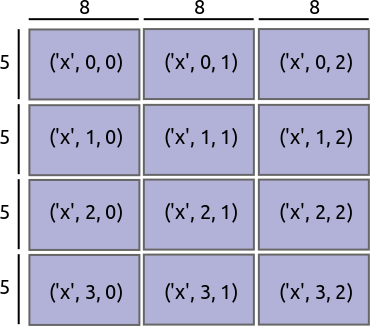• 并行 (Parallel)：使用计算机的所有核
• 大于内存 (Larger-than-memory)：通过将数组分解成许多小块，按顺序对这些块进行操作以最大限度地减少计算的内存占用，并有效地从磁盘流式传输数据，让您可以处理大于可用内存的数据集
• 分块算法 (Blocked Algorithms)：通过执行许多较小的计算来执行大型计算

import matplotlib.pyplot as plt
%matplotlib inline
%config InlineBackend.figure_format='retina'


## 创建数据

%run prep.py -d random


## 设置

from dask.distributed import Client

client = Client(n_workers=8)
client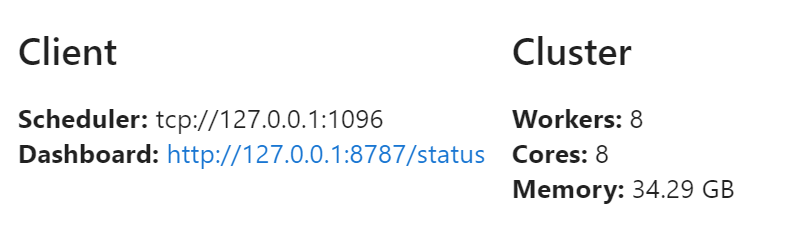## 分块算法

Blocked Algorithms

import h5py
from pathlib import Path

f = h5py.File(Path("data", "random.hdf5"), mode="r")
dset = f["/x"]


1. 计算数组的每个 1,000,000 大小块的总和
2. 计算 1,000 个中间和的总和

%%time
# 计算大数组的总和，一次一百万个数字
sums = []
for i in range(0, 1_000_000_000, 1_000_000):
chunk = dset[i: i + 1_000_000]  # 拉出 numpy 数组
sums.append(chunk.sum())

total = sum(sums)
print(total)

999980528.625
Wall time: 28.5 s


### 练习：使用分块算法计算均值

1. 计算每个块的总和
2. 计算每个块的长度
3. 计算 1,000 个中间和的总和和 1,000 个中间长度的总和，然后除以另一个

# 计算数组均值
sums = []
lens = []
for i in range(0, 1_000_000_000, 1_000_000):
chunk = dset[i: i + 1_000_000]  # 拉出 numpy 数组
lens.append(len(chunk))
sums.append(chunk.sum())

total = sum(sums)
length = sum(lens)
mean = total/length
print(mean)

0.999980528625


## dask.array 包含这些算法

Dask.array 是一个类似 NumPy 的库，它使用这些技巧来对不适合内存的大型数据集进行操作。 它超越上面讨论的线性问题，扩展到完整的 N 维算法和 NumPy 接口的一个不错的子集。

1. data：任何支持 NumPy 切片的对象，例如 dset
2. chunks：告诉我们如何分组数组的块大小，例如 (1_000_000,)
import dask.array as da
x = da.from_array(dset, chunks=(1_000_000,))
x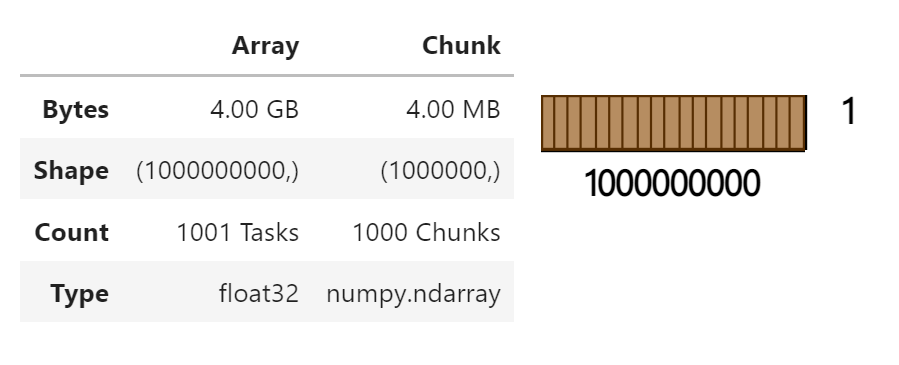dask_array.sum() 创建计算的表达式。 它还没有进行计算。 numpy_array.sum() 立即计算总和。

Dask 数组被分成块。 每个块都必须显式地在该块上运行计算。 如果所需的答案来自整个数据集的一小部分，则在所有数据上运行计算将浪费 CPU 和内存。

result = x.sum()
result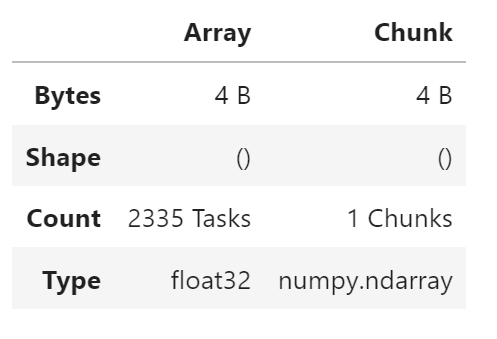Dask.array 对象延迟评估。 .sum 之类的操作构建了一个要执行的分块任务图。

result.compute()

999980540.0


### 练习：计算均值

result = x.mean()
result.compute()

0.99998057


## 性能和并行性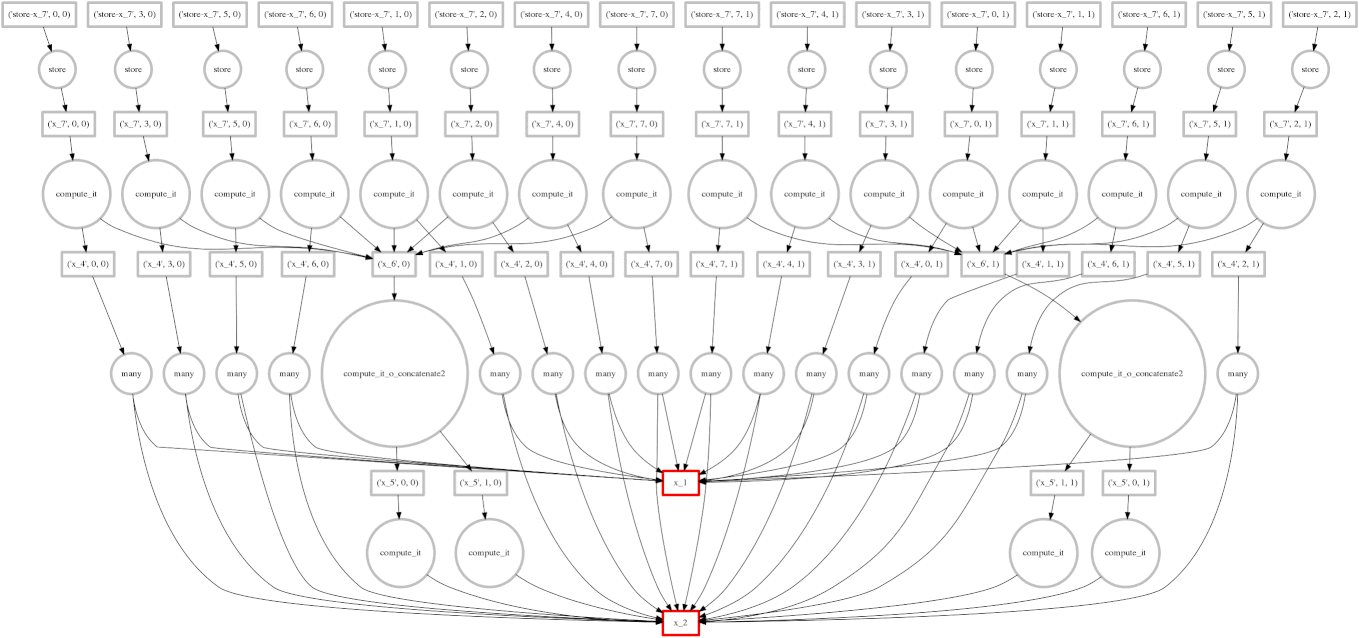1. 并行使用多个内核
2. 在移动到下一个块之前对单个块进行链式操作

Dask.array 将您的数组操作转换为相互关联的任务图，它们之间具有数据依赖关系。 然后 Dask 使用多个线程并行执行此图。 我们将在下一节中详细讨论这一点。

### 示例

1. 构建一个 20000x20000 的正态分布随机值数组，该数组被分成 1000x1000 大小的块
2. 沿一个轴取平均值
3. 每 100 个元素取一个值
import numpy as np

x = da.random.normal(
10, 0.1,
size=(20_000, 20_000),  # 4亿个元素数组
chunks=(1_000, 1_000)   # 切成 1000x1000 大小的块
)
y = x.mean(axis=0)[::100]   # 执行 NumPy 风格的操作


x.nbytes / 1e9

3.2


%%time

y.compute()

Wall time: 3.69 s
array([ 9.9983555 ,  9.99995829,  9.99950296,  9.99986182,  9.99982619,
...
10.00020971,  9.99867086, 10.00015668, 10.00062269,  9.99945275])


## 性能比较

### NumPy

import numpy as np

%%time
x = np.random.normal(10, 0.1, size=(20000, 20000))
y = x.mean(axis=0)[::100]
y

Wall time: 11.6 s
array([10.00080417,  9.9999597 , 10.00110176,  9.9996947 , 10.0015106 ,
...
9.99995008,  9.99979497,  9.99997799, 10.00009926, 10.00007763])


import dask.array as da

%%time
x = da.random.normal(
10, 0.1,
size=(20000, 20000),
chunks=(1000, 1000)
)
y = x.mean(axis=0)[::100]
y.compute()

Wall time: 3.57 s
array([10.00021221, 10.00003971,  9.99979332, 10.0003238 , 10.0005408 ,
...
9.99963974,  9.99982947,  9.99990702, 10.00023817,  9.99974663])


### 讨论

• 计算会在 4 秒内运行吗？
• 将使用多少内存？
• CPU和内存会发生什么？

### 练习：气象数据

data/weather-big/*.hdf5 的 HDF5 文件中有 2GB 有点儿人为的天气数据。 我们将使用 h5py 库与数据进行交互，并使用 dask.array 计算。

1. 为磁盘上数据 (dsets) 每天创建一个 h5py.Dataset 对象
2. 使用 da.from_array 调用包装这些对象
3. 使用 da.stack 在时间维度上堆叠数据集
4. 使用 .method() 方法沿新堆叠成的时间维度计算均值
5. 使用 matplotlib.pyplot.imshow 可视化结果

%run prep.py -d weather

import h5py
from pathlib import Path

filenames = sorted(Path("data", "weather-big").glob("*.hdf5"))
dsets = [h5py.File(filename, mode="r")["/t2m"] for filename in filenames]
dsets

<HDF5 dataset "t2m": shape (5760, 11520), type "<f8">


dsets[::5, ::5]

array([[22.53240967, 29.3604126 , 36.18841553, ..., 41.65081787,
...
44.03045654, 35.39703369]])

%matplotlib inline
import matplotlib.pyplot as plt

fig = plt.figure(figsize=(16, 8))
plt.imshow(dsets[::4, ::4], cmap="RdBu_r")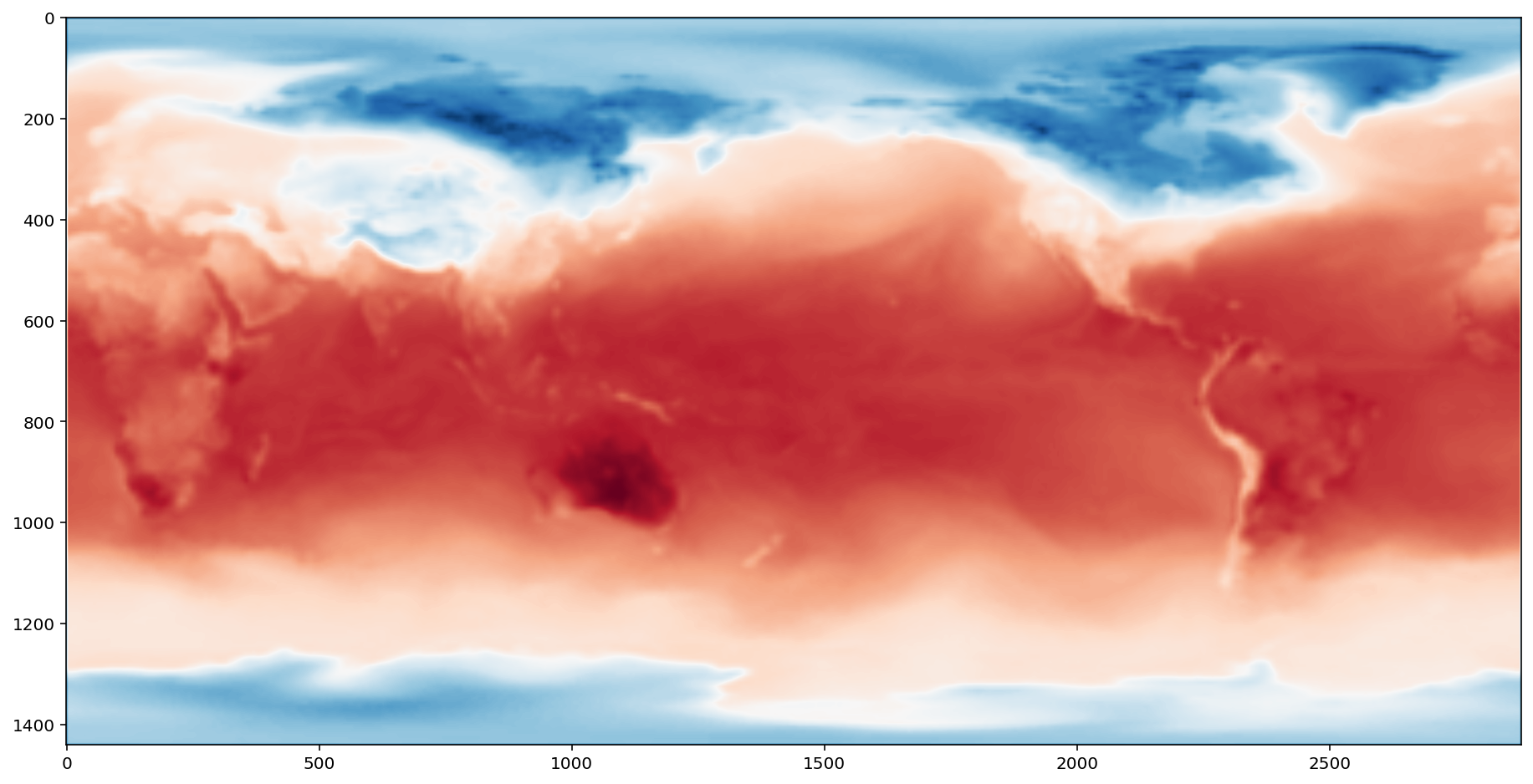dask.array 集成

arrays = [da.from_array(d, chunks=(500, 500)) for d in dsets]
arrays[:2]

[dask.array<array, shape=(5760, 11520), dtype=float64, chunksize=(500, 500), chunktype=numpy.ndarray>,
dask.array<array, shape=(5760, 11520), dtype=float64, chunksize=(500, 500), chunktype=numpy.ndarray>]

arrays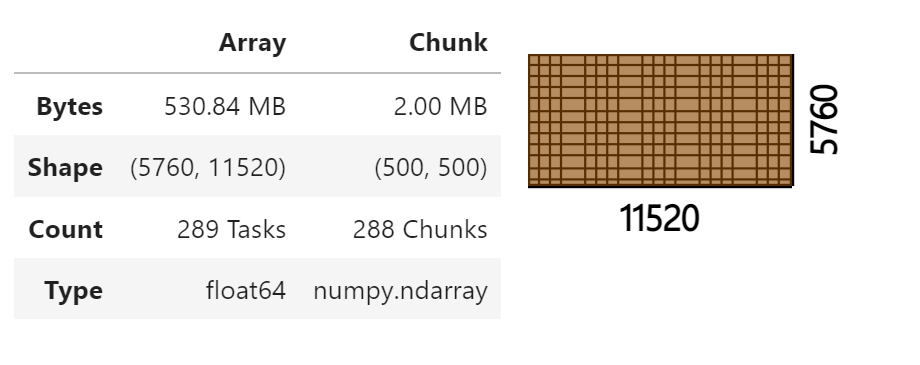x = da.stack(arrays, axis=0)
x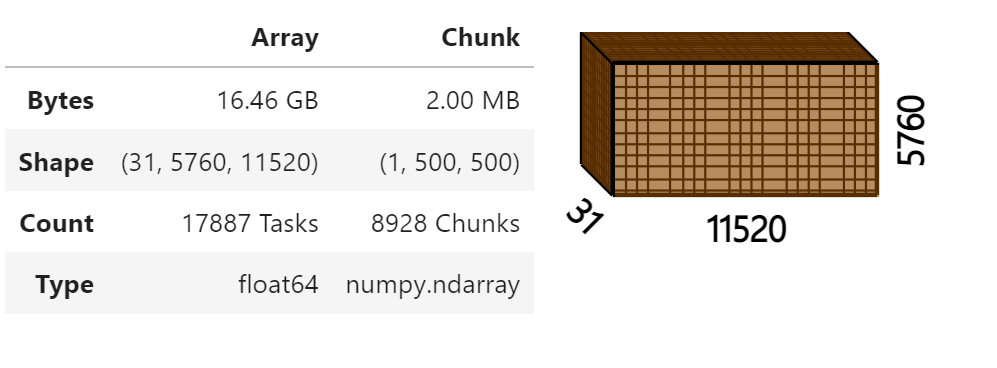result = x.mean(axis=0)
fig = plt.figure(figsize=(16, 8))
plt.imshow(result, cmap="RdBu_r")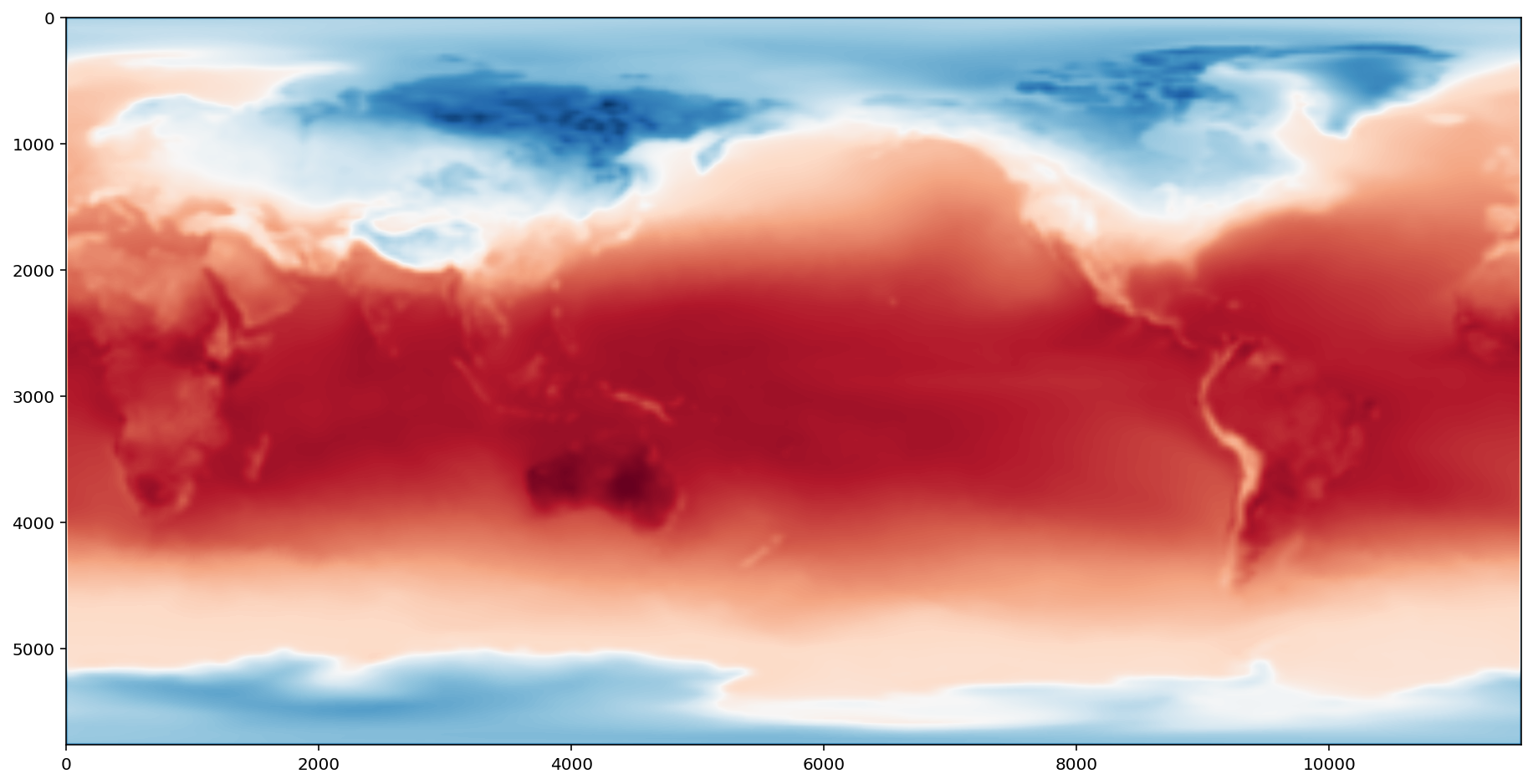result = x - x.mean(axis=0)
fig = plt.figure(figsize=(16, 8))
plt.imshow(result, cmap="RdBu_r")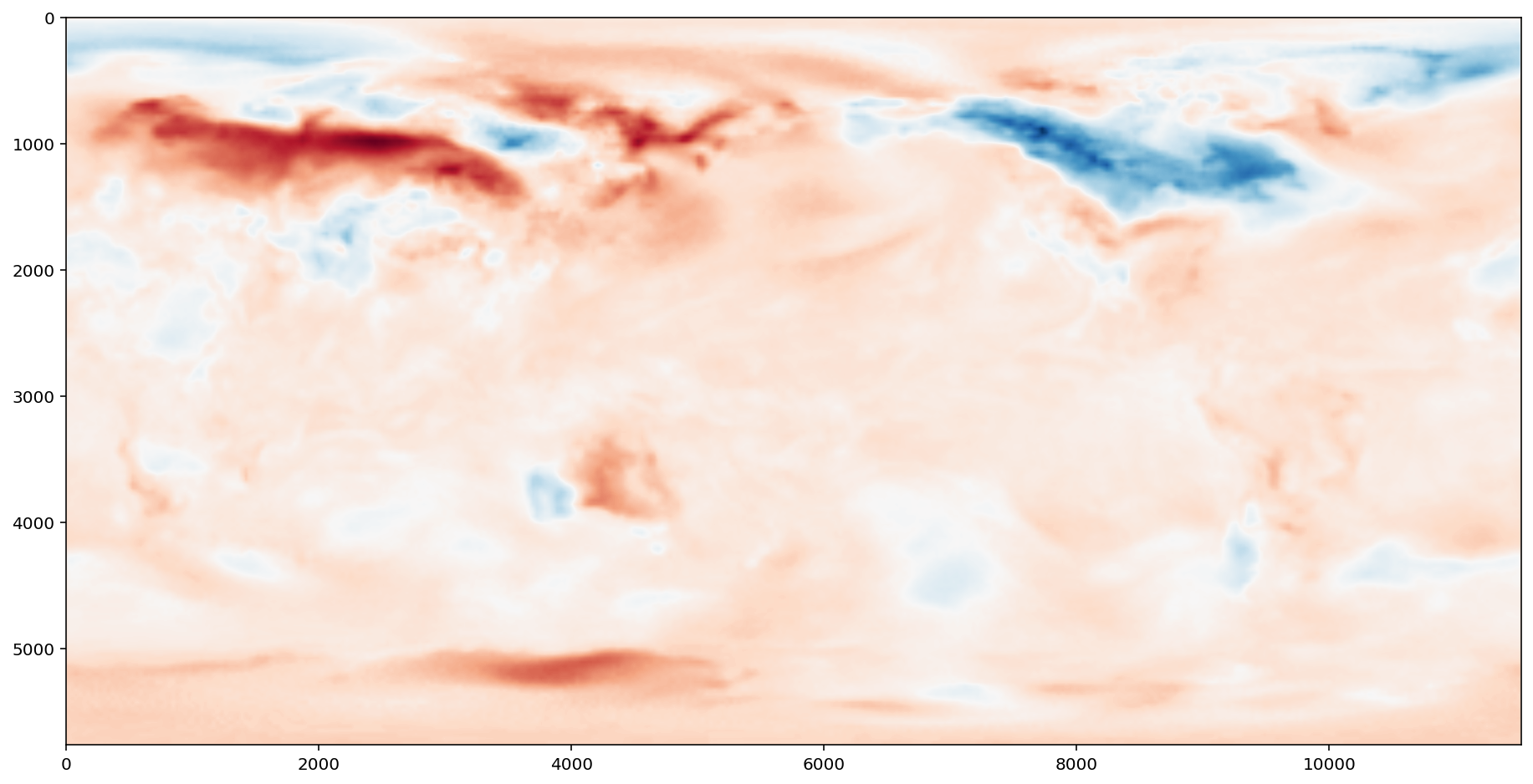### 练习：采样和存储

1. da.store：将 dask.array 存储到任何支持 numpy setitem 语法的对象中，例如
f = h5py.File("myfile.hdf5")
output = f.create_dataset(shape=..., dtype=...)


1. da.to_hdf5：一个专门的函数，用于将一个 dask.array 对象创建并存储到 HDF5 文件中。
da.to_hdf5("data/myfile.hdf5", "/output", my_dask_array)


start:stop:step

>>> L = [1, 2, 3, 4, 5, 6, 7]
>>> L[::3]
[1, 4, 7]

import h5py
from pathlib import Path
import os
filenames = sorted(Path("data", "weather-big").glob("*.hdf5"))
dsets = [
h5py.File(filename, mode="r")["/t2m"]
for filename in filenames
]

arrays = [
da.from_array(dset, chunks=(500, 500))
for dset in dsets
]

x = da.stack(arrays, axis=0)

result = x[:, ::2, ::2]

da.to_zarr(
result,
os.path.join('data', 'myfile.zarr'),
overwrite=True
)


## 练习：Lennard-Jones potential

Lennard-Jones 势 用于物理、化学和工程中的粒子模拟。它是高度可并行化的。

import numpy as np

# 创建粒子随机集合
np.random.seed(seed)
cluster = np.random.normal(0, radius, (notoms, 3)) - 0.5
return cluster

def lj(r2):
sr6 = (1./r2)**3
pot = 4.*(sr6*sr6 - sr6)
return pot

# 创建距离矩阵
def distances(cluster):
diff = cluster[:, np.newaxis, :] - cluster[np.newaxis, :, :]
mat = (diff*diff).sum(-1)
return mat

# 在移除接近零的距离后，在上三角形上计算 lj 函数
def potential(cluster):
d2 = distances(cluster)
dtri = np.triu(d2)
energy = lj(dtri[dtri > 1e-6]).sum()
return energy

cluster = make_cluster(int(7e3), radius=500)

%time potential(cluster)

Wall time: 4.08 s
-0.21282893668845293


%prun -s tottime potential(cluster)

         35 function calls (34 primitive calls) in 3.956 seconds

Ordered by: internal time

ncalls  tottime  percall  cumtime  percall filename:lineno(function)
1    1.316    1.316    2.082    2.082 <ipython-input-33-b553f5fe9fb4>:15(distances)
1    1.238    1.238    1.238    1.238 <ipython-input-33-b553f5fe9fb4>:9(lj)
2    0.798    0.399    0.798    0.399 {method 'reduce' of 'numpy.ufunc' objects}
1    0.309    0.309    3.875    3.875 <ipython-input-33-b553f5fe9fb4>:21(potential)
2/1    0.143    0.072    0.214    0.214 {built-in method numpy.core._multiarray_umath.implement_array_function}
1    0.080    0.080    3.956    3.956 <string>:1(<module>)
1    0.070    0.070    0.070    0.070 {method 'outer' of 'numpy.ufunc' objects}
1    0.000    0.000    3.956    3.956 {built-in method builtins.exec}
2    0.000    0.000    0.000    0.000 {built-in method numpy.arange}
1    0.000    0.000    0.070    0.070 twodim_base.py:365(tri)
1    0.000    0.000    0.210    0.210 twodim_base.py:470(triu)
2    0.000    0.000    0.798    0.399 {method 'sum' of 'numpy.ndarray' objects}
1    0.000    0.000    0.214    0.214 <__array_function__ internals>:2(triu)
2    0.000    0.000    0.798    0.399 _methods.py:45(_sum)
1    0.000    0.000    0.000    0.000 {method 'astype' of 'numpy.ndarray' objects}
1    0.000    0.000    0.000    0.000 {built-in method numpy.zeros}
1    0.000    0.000    0.140    0.140 <__array_function__ internals>:2(where)
2    0.000    0.000    0.000    0.000 twodim_base.py:31(_min_int)
4    0.000    0.000    0.000    0.000 getlimits.py:538(max)
1    0.000    0.000    0.000    0.000 _asarray.py:110(asanyarray)
2    0.000    0.000    0.000    0.000 getlimits.py:525(min)
1    0.000    0.000    0.000    0.000 {built-in method numpy.array}
1    0.000    0.000    0.000    0.000 multiarray.py:321(where)
1    0.000    0.000    0.000    0.000 twodim_base.py:427(_trilu_dispatcher)
1    0.000    0.000    0.000    0.000 {method 'disable' of '_lsprof.Profiler' objects}


%load_ext snakeviz
%snakeviz potential(cluster)


import dask.array as da

# 计算整个距离矩阵的 potential 并忽略除以零
d2 = distances(cluster)
energy = da.nansum(lj(d2))/2.
return energy


from os import cpu_count

dcluster = da.from_array(
cluster,
chunks=cluster.shape//cpu_count()
)


e = potential_dask(dcluster)
%time e.compute()

Wall time: 1.93 s
-0.21282893668845304


## 限制

1. Dask 并没有实现所有的 np.linalg。这已经由许多优秀的 BLAS/LAPACK 实现完成，并且是众多正在进行的学术研究项目的重点。
3. Dask Array 不会尝试像 sort 这样的操作，这些操作众所周知难以并行执行，并且在非常大的数据上价值会有所降低（您实际上很少需要完整排序）。通常我们会包含并行友好的替代方案，如 topk
4. Dask 开发是由迫在眉睫的需求驱动的，因此许多不太常用的功能，如 np.sometrue 并不是纯粹出于懒惰而实现的。这些将做出出色的社区贡献。
client.shutdown()